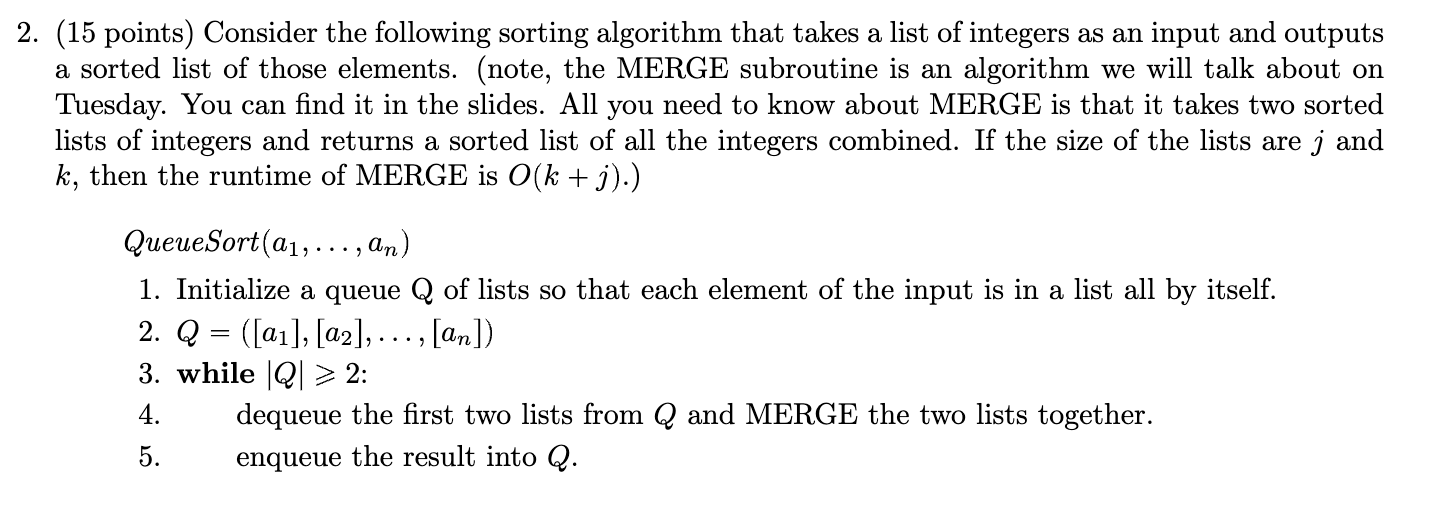Home / Expert Answers / Computer Science / 15-points-consider-the-following-sorting-algorithm-that-takes-a-list-of-integers-as-an-input-and-pa379

# (Solved): (15 points) Consider the following sorting algorithm that takes a list of integers as an input and ...(15 points) Consider the following sorting algorithm that takes a list of integers as an input and outputs a sorted list of those elements. (note, the MERGE subroutine is an algorithm we will talk about on Tuesday. You can find it in the slides. All you need to know about MERGE is that it takes two sorted lists of integers and returns a sorted list of all the integers combined. If the size of the lists are and , then the runtime of MERGE is .) QueueSort 1. Initialize a queue of lists so that each element of the input is in a list all by itself. 2. 3. while : 4. dequeue the first two lists from and MERGE the two lists together. 5. enqueue the result into .

We have an Answer from Expert